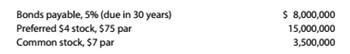Chapter 9, Problem 9.20E

Chapter
Section
Textbook Problem

Six metrics The balance sheet for Shryer Industries Inc. at the end of 20Y9 indicated the following:Income before income tax was $3.400.000, and income taxes were$1,000,000 for the current year. Cash dividends paid on common stock during the current year totaled $100,000. The common stock was selling for$8 per share at the end of the year. Deter mine each of the following: (a) times interest earned, (b) times preferred dividends earned, (c) earnings per share on common stock, (d) price-earnings ratio, (e) dividends per share of common stock, and (f) dividend yield. Round to one decimal place except earnings per share and dividends per share, which should be rounded 10 the nearest cent.

To determine

(a)

Introduction:

Accounting ratios are used to evaluate the financial performance of the business organisation.

Time interest earned TIE :

It is a matric used to measure a company's ability to meet debit obligation it can be calculated using following formula:

Time interest earned=Earning before interest and taxes  Total Interest payable

The time interest earned.

Explanation

Time interest earned=Earning before interest and taxes  Total Interest payable

To determine

(b)

Concept Introduction:

Time preferred dividends earned: it measures company's ability to pay preferred dividends in it also known as dividends coverage it can be calculated using following formula:

Time preferred dividends earned=Net income  Prefrential dividends payout

To calculate:

Time preferred dividends earned

To determine

(c)

Concept Introduction:

Earnings per share: show how many dollars of net income is earned by common stockholder, it can be calculated using following formula.

Earnings per share=Net income  common stock outstanding

To calculate:

The profit earned on each issued share using earning per share.

To determine

(d)

Concept Introduction:

Price earnings ratio P/E ratio: it indicates the amount an investor can expect to invest in a company in order to receive one dollar of company's earnings.

Price earning ratio=Market value of share Earning per share

Expected price of share based on earning using price earnings ratio.

To determine

(e)

Concept Introduction:Dividends per share:

It is the sum of ordinary dividends declared by the company.

Dividends per share=total dividends payout Out standing shares

Dividends per share of common stock.

To determine

(f)

Concept Introduction:

Dividends yield: is an estimation of dividend only return of stock investment.

Dividends yield=Annual dividendshare price

To calculate:

Annual dividends received per share.

Still sussing out bartleby?

Check out a sample textbook solution.

See a sample solution

The Solution to Your Study Problems

Bartleby provides explanations to thousands of textbook problems written by our experts, many with advanced degrees!

Get Started

What is the purpose of a work sheet?

College Accounting (Book Only): A Career Approach

What does the invisible hand of the marketplace do?

Essentials of Economics (MindTap Course List)

EVALUATING RISK AND RETURN Bartman Industriess and Reynolds Inc.s stock prices and dividends, along with the Wi...

Fundamentals of Financial Management, Concise Edition (with Thomson ONE - Business School Edition, 1 term (6 months) Printed Access Card) (MindTap Course List)

What is Miamis living wage rate?

PAYROLL ACCT.,2019 ED.(LL)-TEXT